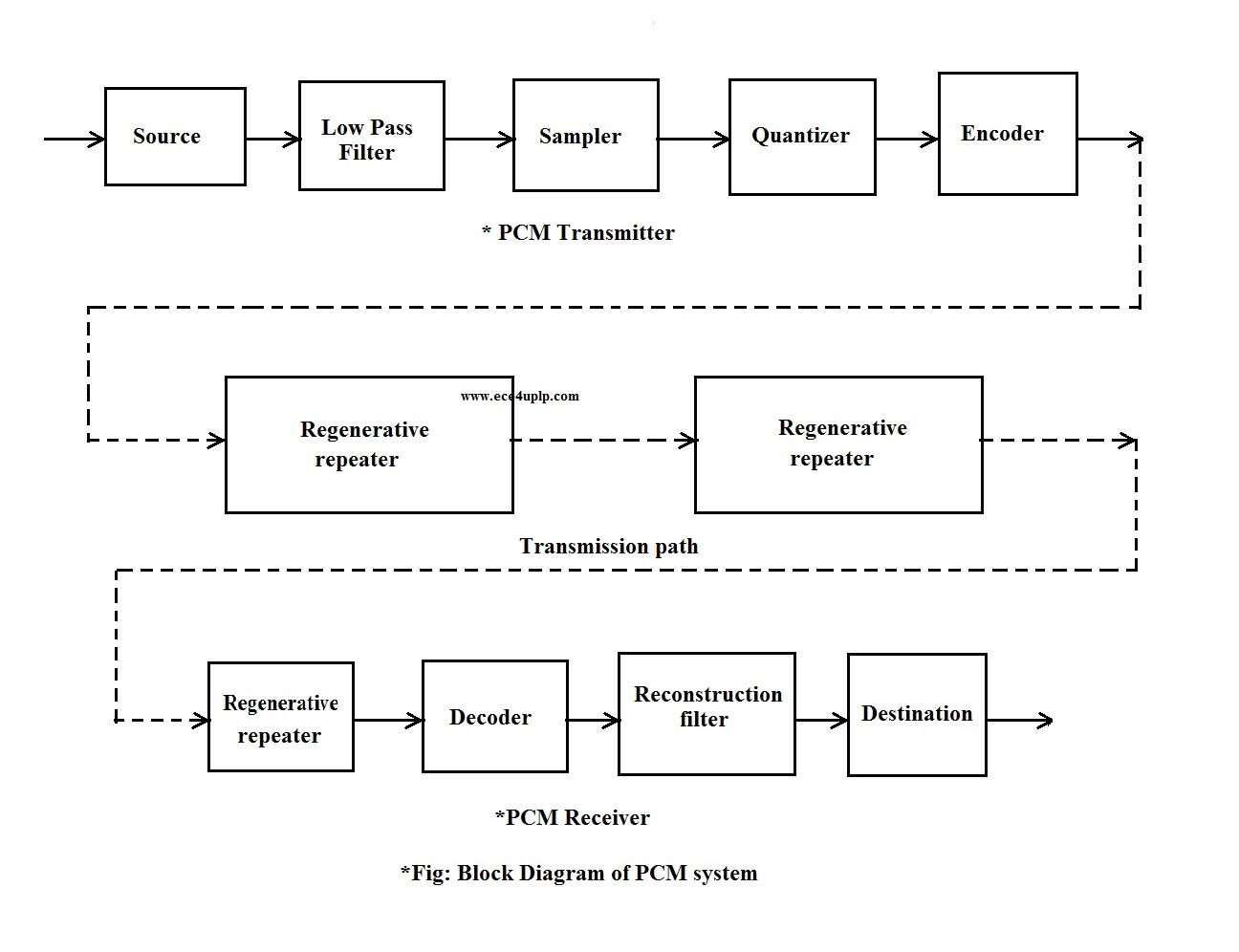## ece4uplp

Amplitude Shift Keying (ASK) (or) On-Off Keying (OOK) is the simplest Digital Modulation technique.

In this method, carrier amplitude is switched between two voltages ON and OFF levels depending upon the input binary sequence.

The carrier signal is a continuous wave (or) sinusoidal waveform.

The normalized power is.

The carrier signal can be expresses in terms of power as.

if energy per bit isand the bit interval asthen the carrier signal is.

Now according to ASK Binary ‘1’ is represented with carrier voltage and Binary ‘0’ is represented with zero voltage.in terms of Energy and bit duration ASK signal can be written as.

It is a simple product Modulator, which modulates the incoming binary sequence (in the form of a signal) with the carrier signal S(t)

i.e,b(t) represents the binary sequence in the form of a signal.

when the  input bit (or) symbol is Binary ‘1’ product Modulator passes the carrier signal and for Binary’0′, A zero output is given which blocks the carrier signal..

The figure shows the Block Diagram of coherent ASK/BASK Detector. The ASK signalis applied to the correlator ( The Block product Modulator followed up by the Integrator).is multiplied by local carrier C(t) this carrier C(t) is phase locked with that of the carrier used in the Transmitter. As this is coherent reception.

The productis applied to the Integrator. The Integrator integrates the input over one bit intervaland the output is given to a threshold device. If the threshold voltage is set to 0 V.

the output of threshold device v(t) (or) v is either ‘1’ (or) ‘0’ based on the following condition.Note:- The input to demodulator is notalways most of the times it is interfered with noise n(t) in the channel.

in coherent detection input to the demodulator is simplysignal where as in Non-coherent detection the input is noisy ASK signal.

Note: There is a rating embedded within this post, please visit this post to rate it.

## Pulse Code Modulation (PCM)

After getting an idea about the sampling & Quantization process we are now ready to describe pulse code modulation (PCM)

PCM is the most basic form of digital pulse modulation.

PCM:- In PCM a message signal is represented by a sequence of coded pulses. which is accomplished by representing the signal in discrete form in both time and amplitude.

PCM Block Diagram:-

The Block Diagram of a PCM system is given in the following figure. This Block Diagram consists of the Basic elements of a PCM system.i. Transmitter

ii. Transmission path.

PCM Transmitter:-  The basic operations performed in the transmitter are

• Sampling
• Quantization
• Coding

is known as Analog-to-Digital conversion (ADC).

LPF:- The LPF prior to the sampler is inclined to prevent the aliasing of the message signal.

Sampler:- Converts the analog Band limited Signal to a discrete signal.

ADC:- It’s a device that performs two operations known as Quantization and Encoding.

Regenerative repeater:- PCM encoded output is transmitted through the transmission path to the receiver, during transmission the PCM output get distorted in the channel by noise which can be generated by the device called regenerative repeater.

PCM Receiver:- The essential operations in the receiver are regeneration of impaired PCM output decoding.

The device that performs decoding and demodulation at the Receiver is known as (DAC) Digital-to-Analog Converter.